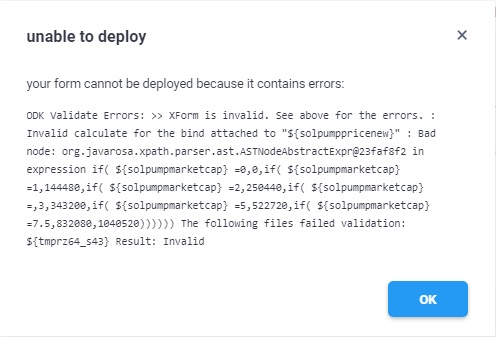# Error message in if statement

Hello,
I am trying to use following formula
if(\${pump_HP_Sol}=0,0,if(\${pump_HP_Sol}<=1,1,if(\${pump_HP_Sol}<=2,2,if(\${pump_HP_Sol}<=3,3,if(\${pump_HP_Sol}<=5,5,if(\${pump_HP_Sol}<=7.5,7.5,10))))))

If used individually in a separate file this formula works fine but when used in actual project i am getting following error messageAny suggestions?

Regards,
Mandar

@mandarsathe, could you try to list out your dummy questions and choices for this so that we understand it clearly. At the moment I see that you are trying to use a condition that meets all the if condition you have stated i.e. your condition `<=7.5` satisfies `<=5.5` which also satisfies `<=3.3`, `<=2.2` and `<=1.1`.

In original project, this formula was linked to another formula. There was an additional “,” in this another formula and thus was the error message. The model is working now.
Thanks for your support @Kal_Lam .

– Mandar

1 Like

Thank you for confirming, @mandarsathe!